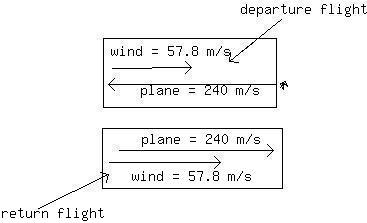# A jetliner can fly 6.00 hours on a full load of fuel. Without wind it flies at a speed of 2.40 X 102m/s. The planes to make a round-trip by heading dunaivlingr 2020-12-06 Answered
A jetliner can fly 6.00 hours on a full load of fuel. Without wind it flies at a speed of 2.40 X 102m/s. The planes to make a round-trip by heading due west for a certain distance,turning around, and then heading due east for the return trip.During the entire flight, however, the place encounters a 57.8 m/s wind from the jet stream, which blows from west to east. What is the maximum distance that the plane can travel due west and just be able to return home ?
You can still ask an expert for help

• Questions are typically answered in as fast as 30 minutes

Solve your problem for the price of one coffee

• Math expert for every subject
• Pay only if we can solve itdieseisB

The jetliner can fly for 6 hrs on a load of fuel, so the whole trip would have to take 6 hours.
So that is
=21600 seconds it can fly
and at is how far the plane can travel in the round trip. let's see a diagram:you can see that the wind counteracts the velocity of the plane on the departure flight so the velocity of the plane will be $\left(240-57.8\right)=182.2m/s$
on the way back, the wind and plane velocity will add up sothe total velocity will be $\left(240+57.8\right)=297.8m/s$
so we can say:
so on the way back the plane travels $\left(297.8-182.2\right)=115.6$ m/sfaster than on the departure.
the distance both ways must be the same so:
distance there $=182.2$ $\frac{m}{s}\cdot {t}_{1}$
distance back $=297.8$ $\frac{m}{s}\cdot {t}_{2}$
for every
if we take the ratio of these 2 : $\frac{297.8}{182.2}$
$=1.6345$ how many times faster the return time isthat the departure.
${t}_{1}=1.6345{t}_{2}$. the total time (${t}_{1}+{t}_{2}$ must be 6 hrs or 21 600 seconds)
so substitute in the above to get:
$\left(1.6345{t}_{2}\right)+{t}_{2}=21600$ seconds.
$2.6345{t}_{2}=21600$ s
${t}_{2}=8198.899$ seconds.
so ${t}_{1}$ must be $21600-8198.899=13401.1$ seconds. now we can plug this into our distane formula above:
distance there is the distance that the plance can travel